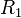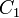Errata: In Example 5.8, the third nodal equation should be deleted

In Example 5.8, only two nodal equations are required: one for node betweenandand one for the node at theinput to the op amp. The correct equations are: